# GSEB Solutions Class 12 Maths Chapter 11 Three Dimensional Geometry Miscellaneous Exercise

Gujarat Board GSEB Textbook Solutions Class 12 Maths Chapter 11 Three Dimensional Geometry Miscellaneous Exercise Textbook Questions and Answers.

## Gujarat Board Textbook Solutions Class 12 Maths Chapter 11 Three Dimensional Geometry Miscellaneous Exercise

Question 1.
Show that the line joining the origin to the point (2, 1, 1) is perpendicular the line determined by the points (3, 5, – 1) and (4, 3, – 1).
Solution:
First two points A and B are (0, 0, 0) and (2, 1, 1) respectively.
∴ Direction ratios of AB are 2, 1, 1.
Direction ratios of CD joining the points C(3, 5, – 1) and D(4, 3, – 1) are (1, – 2, 0)
If AB ⊥ CD, then a1.a2 + b1.b2 + c1.c2 = 0
i.e., 2.1 + 1.(- 2) + 1.0
= 2 – 2 + 0 = 0,
which is true.
⇒ AB is perpendicular to CD.Question 2.
If l1, m1, n1 and l2, m2, n2 are the direction cosines of two mutually perpendicular lines,
show that the directions cosines of the line perpendicular to both of these are m1n2 – m2n1, n1l2 – n2l1, l1m2 – l2m1.
Solution:
l1, m1, n1 and l2, m2, n2 are the direction cosines of two mutually perpendicular lines.
Therefore l1l2 + m1m2 + n1n2 = 0 …………….. (1)
Also, $$l_{1}^{2}$$ + $$m_{1}^{2}$$ + $$n_{1}^{2}$$ = 1
and $$l_{2}^{2}$$ + $$m_{2}^{2}$$ + $$n_{2}^{2}$$ = 1 ………………… (2)
Let l, m, n be the direction cosines of the line perpendicular to the lines with direction cosines l1, m1, n1 and l2, m2, n2.
⇒ ll1 + mm1 + nn1 = 0
and ll2 + mm2 + nn2 = 0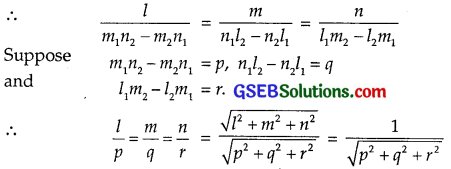Now consider the identity
($$l_{1}^{2}$$ + $$m_{1}^{2}$$ + $$n_{1}^{2}$$)($$l_{2}^{2}$$ + $$m_{2}^{2}$$ + $$n_{2}^{2}$$) – (l1l2 + m1m2 + n1n2)2.
= (m1n2 – m2n1)2 + (n1l2 – n2l1)2 + (l1m2 – l2m1)2
R.H.S. = p2 + q2 + r2
= ($$l_{1}^{2}$$ + $$m_{1}^{2}$$ + $$n_{1}^{2}$$)($$l_{2}^{2}$$ + m$$m_{2}^{2}$$ + $$n_{2}^{2}$$) – (l1 l2 + m1m2 + n1n2)2
From (1) and (2)
l1l2 + m1m2 + n1n2 = 0
and $$l_{1}^{2}$$ + $$m_{1}^{2}$$ + $$n_{1}^{2}$$ = 1 and $$l_{2}^{2}$$ + $$m_{2}^{2}$$ + $$n_{2}^{2}$$ = 1
Putting these values in (3), we get
p2 + q2 + r2 = 1.1 – 0 = 1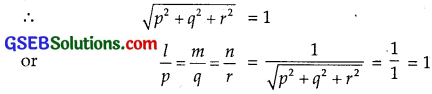⇒ l = p = m1n2 – m2n1, m = q = n1l2 – n2l1
and n = r = l2m2 – l2m1.
Hence, the required direction cosines are
m1n2 – m2n1, n1l2 – n2l1, l1m2 – l2m1.

Question 3.
Find the angle between the lines whose direction ratios are a, b, c and b – c, c – a, a – b.
Solution:
a, b, c and b – c, c – a, a – b are the direction ratios of two lines. The angle between the lines is given byQuestion 4.
Find the equation of the line parallel to x-axis and passing through the origin.
Solution:
The line parallel to x-axis and passing through the origin is x-axis itself.
∴ Equation of x-axis is y = 0, z = 0.

Question 5.
If the coordinates of the points A, B, C and D are (1, 2, 3), (4, 5, 7), (- 4, 3, – 6) and (2, 9, 2) respectively, then find the angle between AB and CD.
Solution:
Direction ratios of AB when A and B are (1, 2, 3) and (4, 5, 7) respectively are 4 – 1, 5 – 2, 7 – 3 or 3, 3, 4.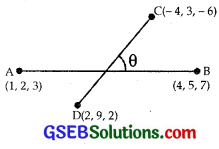Direction ratios of the line joining the points C(- 4, 3, – 6) and D(2, 9, 2) are 2 + 4, 9 – 3, 2 + 6 or 6, 6, 8.
Direction ratios of AB and CD are proportional.
⇒ Angle between these lines is zero.
⇒ The lines AB and CD are parallel.

Question 6.
If the lines $$\frac{x-1}{-3}$$ = $$\frac{y-2}{2k}$$ = $$\frac{z-3}{2}$$
and $$\frac{x-1}{3k}$$ = $$\frac{y-1}{1}$$ = $$\frac{z-6}{-5}$$ are perpendicular, find the value of k.
Solution:
The two lines are
$$\frac{x-1}{-3}$$ = $$\frac{y-2}{2k}$$ = $$\frac{z-3}{2}$$ ……………. (1)
and $$\frac{x-1}{3k}$$ = $$\frac{y-1}{1}$$ = $$\frac{z-6}{-5}$$ ……………. (2)
The direction ratios of the lines (1) and (2) are – 3, 2k, 2 and 3k, 1, – 5 respectively.
Lines (1) and (2) are perpendicular to each other, if
a1a2 + b1b2 + c1c2 = 0
i.e. – 3(3k) + 2k(1) + 2(- 5) = 0
⇒ – 9k + 2k – 10 = 0 or 7k + 10 = 0
∴ k = – $$\frac{10}{7}$$.Question 7.
Find the vector equation of the line passing through (1, 2, 3) and perpendicular to the plane $$\vec{r}$$.($$\hat {i}$$ + 2$$\hat {j}$$ – 5$$\hat {k}$$) + 9 = 0.
Solution:
Direction ratios of the normal of the plane $$\vec{r}$$.($$\hat {i}$$ + 2$$\hat {j}$$ – 5$$\hat {k}$$) + 9 = 0 are 1, 2, – 5.
The equation of line passing through $$\vec{r}_{1}$$ and with direction ratios b1, b2, b3 is
$$\vec{r}$$ = $$\vec{r}_{1}$$ + λ(b1$$\hat {i}$$ + b2$$\hat {j}$$ + b3$$\hat {k}$$)
Hence, the line passing through (1, 2, 3) and having the direction ratios 1, 2, – 5 is
$$\vec{r}$$ = $$\hat {i}$$ + 2$$\hat {j}$$ + 3$$\hat {k}$$ + λ($$\hat {i}$$ + 2$$\hat {j}$$ – 5$$\hat {k}$$).

Question 8.
Find the equation of the plane passing through the point (a, b, c) and parallel to the plane $$\vec{r}$$.($$\hat {i}$$ + $$\hat {j}$$ + $$\hat {k}$$) = 2.
Solution:
Any plane parallel to the plane $$\vec{r}$$.($$\hat {i}$$ + $$\hat {j}$$ + $$\hat {k}$$) = 2 is $$\vec{r}$$.($$\hat {i}$$ + $$\hat {j}$$ + $$\hat {k}$$) = λ.
This plane passes through the point a$$\hat {i}$$ + b$$\hat {j}$$ + c$$\hat {k}$$.
∴ (a$$\hat {i}$$ + b$$\hat {j}$$ + c$$\hat {k}$$).($$\hat {i}$$ + $$\hat {j}$$ + $$\hat {k}$$) = λ
or a + b + c = λ
∴ Equation of the required plane is $$\vec{r}$$.($$\hat {i}$$ + $$\hat {j}$$ + $$\hat {k}$$) = a + b + c.Question 9.
Find the shortest distance between the lines
$$\vec{r}$$ = 6$$\hat {i}$$ + 2$$\hat {j}$$ + 2$$\hat {k}$$ + λ($$\hat {i}$$ – 2$$\hat {j}$$ + 2$$\hat {k}$$)
and $$\vec{r}$$ = – 4$$\hat {i}$$ – $$\hat {k}$$ + µ(3$$\hat {i}$$ – 2$$\hat {j}$$ – 2$$\hat {k}$$).
Solution:
If $$\vec{a}_{1}$$ and $$\vec{a}_{2}$$ are the two points on the lines and $$\vec{b}_{1}$$ and $$\vec{b}_{2}$$
and are their directions then the shortest distance between themThe given lines are
$$\vec{r}$$ = 6$$\hat {i}$$ + 2$$\hat {j}$$ + 2$$\hat {k}$$ + λ($$\hat {i}$$ – 2$$\hat {j}$$ + 2$$\hat {k}$$)
and $$\vec{r}$$ = – 4$$\hat {i}$$ – $$\hat {k}$$ + µ(3$$\hat {i}$$ – 2$$\hat {j}$$ – 2$$\hat {k}$$)
Here, $$\vec{a}_{1}$$ = 6$$\hat {i}$$ + 2$$\hat {j}$$ + 2$$\hat {k}$$, $$\vec{a}_{2}$$ = – 4$$\hat {i}$$ – $$\hat {k}$$
and $$\vec{b}_{1}$$ = $$\hat {i}$$ – 2$$\hat {j}$$ + 2$$\hat {k}$$, $$\vec{b}_{2}$$ = 3$$\hat {i}$$ – 2$$\hat {j}$$ – 2$$\hat {k}$$Question 10.
Find the coordinates of a point, where the line through (5, 1, 6) and (3, 4, 1) cross the YZ – plane.
Solution:
The equations of the line passing through (x1, y1, z1) and (x2, y2, z2) are \The points are (5, 1, 6) and (3, 4, 1).
∴ Equations of the line passing through these points are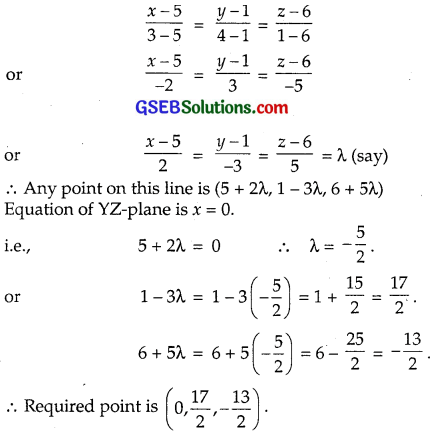Question 11.
Find the coordinates of the point, where the line passing through (5, 1, 6) and (3, 4, 1) crosses ZX – plane.
Solution:
From Q.no. 10, equation of the line passing through (5, 1, 6) and (3, 4, 1)
$$\frac{x-5}{2}$$ = $$\frac{y-1}{-3}$$ = $$\frac{z-6}{5}$$ = λ
∴ Any point on this line is (5 + 2λ, 1 – 3λ, 6 + 5λ).
Equation of ZX-plane is Y = 0.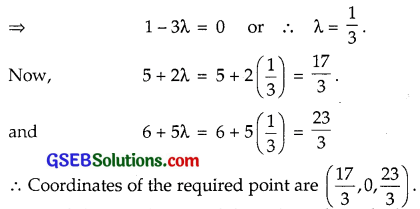Question 12.
Find the coordinates of the point, where the line passing through (3, – 4, – 5) and (2, – 3, 1) crosses the plane 2x + y + z = 7.
Solution:
Equations of the line passing through (x1, y1, z1) and (x2, y2, z2) areThe two points are (3, – 4, – 5) and (2, – 3, 1).
Equations of the line passing through these points are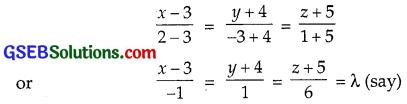∴ Any point on this line is (3 – λ, – 4 + λ, – 5 + 6λ)
The point lies on the plane 2x + y + z = 7.
∴ 2(3 – λ) + (- 4 + λ) + (- 5 + 6λ) = 7
or – 3 + 5λ = 7 ⇒ 5λ = 7 + 3 = 10
∴ λ = 2
⇒ 3 – λ = 3 – 2 = 1,
– 4 + λ = – 4 + 2 = – 2
and – 5 + 6λ = – 5 + 6 × 2 = 7.
∴ The line passing through (3, – 4, – 5) and (2, – 3, 1) crosses the plane 2x + y + z = 7 at (1, – 2, 7).Question 13.
Find the equation of the plane passing through the point (- 1, 3, 2) and perpendicular to each of the planes x + 2y + 3z = 5 and 3x + 3y + z = 0.
Solution:
Equation of the plane passing through (- 1, 3, 2) is
a(x + 1) + b(y – 3) + c(z – 2) = 0 …………… (1)
Plane (1) is perpendicular to the plane x + 2y + 3z = 5.
∴ a. 1 + b. 2 + c. 3 = 0
[When normals are perpendicular a1a2 + b1b2 + c1c2 = 0]
or a + 2b + 3c = 0 ………….. (2)
Further plane (1) is perpendicular to 3x + 3y + z = 0.
∴ a.3 + b.3 + c.1 = 0
or 3a + 3b + c = 0 ………….. (3)
From (2) and (3)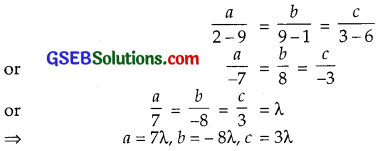Putting these values in (1),
7λ(x + 1) – 8λ(y – 3) + 3λ(z – 2) = 0
or 7(x + 1) – 8(y – 3) + 3(z – 2) = 0
or 7x – 8y + 3z + 7 + 24 – 6 = 0
or 7x – 8y + 3z + 25 = 0
∴ Equation of the required plane is 7x – 8y + 3z + 25 = 0.

Question 14.
If the points (1, 1, p) and (- 3, 0, 1) are equidistant from the plane $$\vec{r}$$.(3$$\hat {i}$$ + 4$$\hat {j}$$ – 12$$\hat {k}$$) + 13 = 0
Solution:
Let the plane be $$\vec{r}$$.$$\hat {n}$$ = d.
∴ Perpendicular distance from $$\vec{a}$$ to this plane = |d – $$\vec{a}$$.$$\hat {n}$$|.
Here, the plane is $$\vec{r}$$.(3$$\hat {i}$$ + 4$$\hat {j}$$ – 12$$\hat {k}$$) + 13 = 0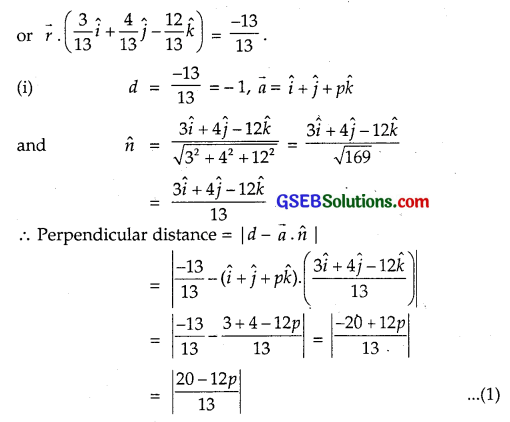The second point is (- 3, 0, 1) or (- 3$$\hat {i}$$ + $$\hat {k}$$).
∴ Perpendicular distance of the plane from the point – 3$$\hat {i}$$ + $$\hat {k}$$ is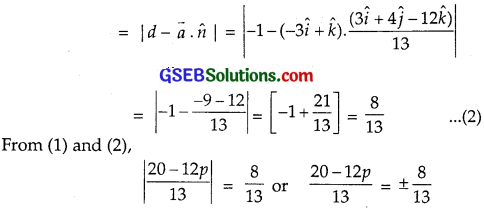or 20 – 12p = ± 8
Taking +ve sign,
20 – 12p = 8
12p = 20 – 8 = 12
∴ p = 1
Taking -ve sign,
20p – 12p = – 8
∴ p = $$\frac{28}{12}$$ = $$\frac{7}{3}$$
∴ p = 1 or $$\frac{7}{3}$$.Question 15.
Find the equation of the plane passing through the line of intersection of the planes
$$\vec{r}$$.($$\hat {i}$$ + $$\hat {j}$$ + $$\hat {k}$$) = 1
and $$\vec{r}$$.(2$$\hat {i}$$ + 3$$\hat {j}$$ – $$\hat {k}$$) + 4 = 0 and parallel to x-axis.
Solution:
The two given planes are
$$\vec{r}$$.(2$$\hat {i}$$ + 3$$\hat {j}$$ – $$\hat {k}$$) + 4 = 0
and $$\vec{r}$$.($$\hat {i}$$ + $$\hat {j}$$ + $$\hat {k}$$) – 1 = 0
∴ Equation of the plane passing through the line of intersection of these planes is
$$\vec{r}$$.(2$$\hat {i}$$ + 3$$\hat {j}$$ – $$\hat {k}$$) + 4 + λ[$$\vec{r}$$.($$\hat {i}$$ + $$\hat {j}$$ + $$\hat {k}$$ – 1] = 0
or $$\vec{r}$$.[(2 + λ)$$\hat {i}$$ + (3 + λ)$$\hat {j}$$ + (- 1 + λ)$$\hat {k}$$] + 4 – λ = 0 ……………. (1)
Also, x-axis is parallel to the plane (1).
⇒ Its normal is perpendicular to x-axis.
Direction ratios of normal are 2 + λ, 3 + λ, – 1 + λ,
Direction ratios of x-axis are 1, 0, 0.
Normal of plane and x-axis being perpendicular, therefore
(2 + λ) × 1 + (3 + λ) × 0 + (- 1 + λ) × 0 = 0
or 2 + λ = 0 ⇒ λ = – 2.
Putting λ = – 2 in (1), we get
$$\vec{r}$$.($$\hat {j}$$ – 3$$\hat {k}$$) + 6 = 0.

Question 16.
If O is the origin and the coordinates of P are (1, 2, – 3) then find the equation of the plane passing through P and perpendicular to OP.
Solution:
The points O and P are (0, 0, 0) and (1, 2, – 3) respectively.
∴ Direction ratios of OP are
1 – 0, 2 – 0, – 3 – 0 or 1, 2, – 3.
The plane passing through (x1, y1, z1) is
a(x – x1) + b(y – y1) + c(z – z1) = 0,
where a, b and c are the direction ratios of the normal.
Here, the direction ratios of normal are 1, 2, – 3 and the point P is (1, 2, – 3).
∴ Equation of the required plane is
1.(x – 1) + 2(y – 2) – 3(z + 3) = 0
or x – 1 + 2y – 4 – 3z – 9 = 0
or x + 2y – 3z – 14 = 0.Question 17.
Find the equation of the plane which contains the line of intersection of the planes $$\vec{r}$$.($$\hat {i}$$ + 2$$\hat {j}$$ + 3$$\hat {k}$$) – 4 = 0
and $$\vec{r}$$.(2$$\hat {i}$$ + $$\hat {j}$$ – $$\hat {k}$$) + 5 = 0 and which is perpendicular to the plane
$$\vec{r}$$.(5$$\hat {i}$$ + 3$$\hat {j}$$ – 6$$\hat {k}$$) + 8 = 0.
Solution:
The two given planes are
$$\vec{r}$$.($$\hat {i}$$ + 2$$\hat {j}$$ + 3$$\hat {k}$$) – 4 = 0 …………….. (1)
and $$\vec{r}$$.(2$$\hat {i}$$ + $$\hat {j}$$ – $$\hat {k}$$) + 5 = 0 …………….. (2)
A plane which contains the line of intersection of the planes (1) and (2) is
$$\vec{r}$$.($$\hat {i}$$ + 2$$\hat {j}$$ + 3$$\hat {k}$$) + 4 + λ[$$\vec{r}$$.(2$$\hat {i}$$ + $$\hat {j}$$ – $$\hat {k}$$) + 5] = 0
or $$\vec{r}$$.[(1 + 2λ)$$\hat {i}$$ + (2 + λ)$$\hat {j}$$ + (3 – λ)$$\hat {k}$$] – 4 + 5λ = 0 …………….. (3)
Now, the plane (3) is perpendicular to the plane
$$\vec{r}$$.(5$$\hat {i}$$ + 3$$\hat {j}$$ – 6$$\hat {k}$$) + 8 = 0 …………….. (4)
⇒ (1 + 2λ) × 5 + (2 + λ) × 3 + (3 – λ) × (- 6) = 0
or 5 + 10λ + 6 + 3λ – 18 + 6λ = 0
or 19λ – 7 = 0
⇒ λ = $$\frac{7}{19}$$.
Putting the value of λ in (3),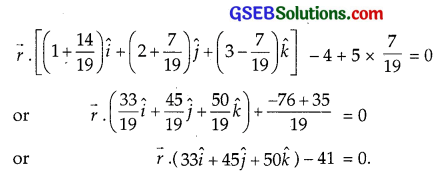This is the required plane.Question 18.
Find the distance of the point (- 1, – 5, – 10) from the point of intersection of the line $$\vec{r}$$ = 2$$\hat {i}$$ – $$\hat {j}$$ + 2k + λ(3$$\hat {i}$$ + 4$$\hat {j}$$ + 2$$\hat {k}$$)
and the plane $$\vec{r}$$.($$\hat {i}$$ – $$\hat {j}$$ + $$\hat {k}$$ = 5.
Solution:
The line and the plane are respectively
$$\vec{r}$$ = 2$$\hat {i}$$ – $$\hat {j}$$ + 2k + λ(3$$\hat {i}$$ + 4$$\hat {j}$$ + 2$$\hat {k}$$) ………………. (1)
and $$\vec{r}$$.($$\hat {i}$$ – $$\hat {j}$$ + $$\hat {k}$$) = 5 …………….. (2)
Solving the equations (1) and (2), we get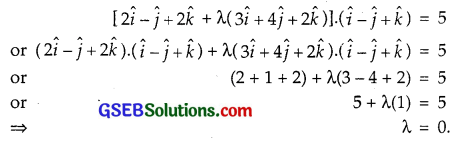∴ The point of intersection of line and the plane is (2$$\hat {i}$$ – $$\hat {j}$$ + 2$$\hat {k}$$).
The other point is (- 1, – 5, – 10)
= – $$\hat {i}$$ – 5$$\hat {j}$$ – 10$$\hat {k}$$.
Distance between these pointsQuestion 19.
Find the vector equation of the line passing through point (1, 2, 3) and parallel to the planes $$\vec{r}$$.($$\hat {i}$$ – $$\hat {j}$$ + 2$$\hat {k}$$) = 5
and $$\vec{r}$$.(3$$\hat {i}$$ + $$\hat {j}$$ + $$\hat {k}$$) = 6.
Solution:
Let the direction of the line be $$\vec{b}$$ = b1$$\hat {i}$$ + b2$$\hat {j}$$ + b3$$\hat {k}$$.
∴ Equation of the line passing through (1, 2, 3) having the directon $$\vec{b}$$ is
$$\vec{r}$$ = $$\hat {i}$$ + 2$$\hat {j}$$ + 3$$\hat {k}$$ + λ$$\vec{b}$$
= $$\hat {i}$$ + 2$$\hat {j}$$ + 3$$\hat {k}$$ + λ(b1$$\hat {i}$$ + b2$$\hat {j}$$ + b3$$\hat {k}$$ ……….. (1)
Line (1) and the plane $$\vec{r}$$.($$\hat {i}$$ – $$\hat {j}$$ + 2$$\hat {k}$$) = 5 are parallel.
⇒ Normal of plane is perpendicular to the line (1).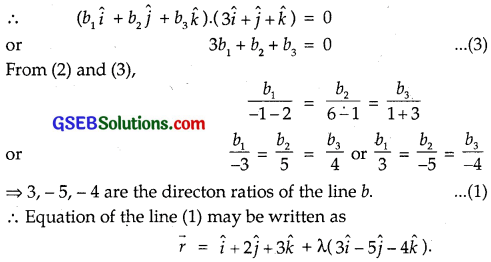Question 20.
Find the vector equation of the line passing through the point (1, 2, – 4) and perpendicular to the two lines $$\frac{x-8}{3}$$ = $$\frac{y+19}{-16}$$
= $$\frac{z-10}{7}$$ and $$\frac{x-15}{3}$$ = $$\frac{y-29}{8}$$ = $$\frac{z-5}{-5}$$.
Solution:
The equation of the line passing through the point (1, 2, – 4) isThe lines (1) and (4) are perpendicular to each other.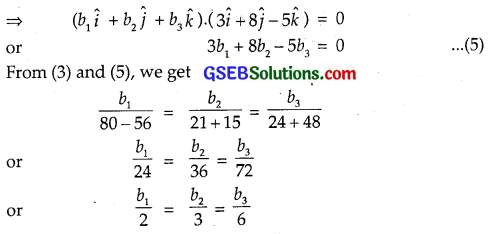∴ Direction of line (1) is 2$$\hat {i}$$ + 3$$\hat {j}$$ + 6$$\hat {k}$$.
∴ Equation of the line is
$$\vec{r}$$ = $$\hat {i}$$ + 2$$\hat {j}$$ – 4$$\hat {k}$$ + λ(2$$\hat {i}$$ + 3$$\hat {j}$$ + 6$$\hat {k}$$).Question 21.
Prove that if a plane has the intercepts a, b and c on the axes and is at a distance p units from the origin, then
$$\frac{1}{a^{2}}$$ + $$\frac{1}{b^{2}}$$ + $$\frac{1}{c^{2}}$$ = $$\frac{1}{p^{2}}$$.
Solution:
Equation of the plane with intercepts a, b and c on axes is
$$\frac{x}{a}$$ + $$\frac{y}{b}$$ + $$\frac{z}{c}$$ = 1.
∴ The perpendicular distance of the plane from the origin is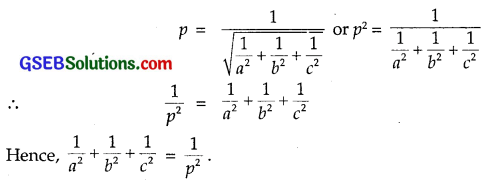Choose the correct answers in questions 22 and 23:

Question 22.
Distance between the planes
2x + 3y + 4z = 4 and 4x + 6y + 8z = 12 is
(A) 2 units
(B) 4 units
(C) 8 units
(D) $$\frac{2}{\sqrt{29}}$$ units
Solution:
Equations of the planes are:
2x + 3y + 4z = 4 ………….. (1)
and 4x + 6y + 8z = 12 ……………. (2)
Dividing equation (2) by 2
2x + 3y + 4z = 6 ……………….. (3)
The distance between parallel lines
ax + by + cz = d1 and ax + by + cz = d2 is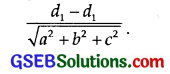∴ The distance between the planes (1) and (3) is∴ Part (D) is the correct answer.Question 23.
The planes are 2x – y + 4z = 5 and 5x – 2.5y + 10z = 6
(A) are perpendicular
(B) are parallel
(C) intersect y-axis
(D) passes through (0, 0, $$\frac{5}{4}$$)
Solution:
The planes are
2x – y + 4z = 5 ……………….. (1)
and 5x – 2.5y + 10z = 6 ………………… (2)
Comparing the coefficients of x, y and z, we get
$$\frac{2}{5}$$ = $$\frac{-1}{-2.5}$$ = $$\frac{4}{10}$$ ………….. (3)
The plánes a1x + b1y + c1z = d1 and a2x + b2y + c2z = d2 are parallel, if
$$\frac{a_{1}}{a_{2}}=\frac{b_{1}}{b_{2}}=\frac{c_{1}}{c_{2}}$$
From (3), this condition is satisfied.
Hence, planes (1) and (2) are parallel.
∴ Part (B) is the correct answer.